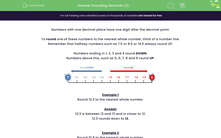# Practise Rounding Decimals to the Nearest Whole Number

In this worksheet, students will round numbers with one decimal place to the nearest whole number. Numbers up to 100 are used.Key stage:  KS 2

Curriculum topic:   Number: Fractions and Decimals

Curriculum subtopic:   Round One Decimal Place Up/Down

Difficulty level:#### Worksheet Overview

Numbers with one decimal place have one digit after the decimal point.

To round one of these numbers to the nearest whole number, think of a number line.

Remember that halfway numbers such as 7.5 or 8.5 or 14.5 always round up.

Numbers ending in 1, 2, 3 and 4 round down.

Numbers above this, such as 5, 6, 7, 8 and 9 round up.Example 1

Round 12.3 to the nearest whole number.

12.3 is between 12 and 13 and is closer to 12.

12.3 rounds down to 12.Example 2

Round 10.8 to the nearest whole number.

10.8 is between 10 and 11 and is closer to 11.

10.8 rounds up to 11.

Example 3

Round 16.5 to the nearest whole number.

16.5 is halfway between 16 and 17 and so we round up.

16.5 rounds up to 17.

Are you happy with all that?Let's get started.

### What is EdPlace?

We're your National Curriculum aligned online education content provider helping each child succeed in English, maths and science from year 1 to GCSE. With an EdPlace account you’ll be able to track and measure progress, helping each child achieve their best. We build confidence and attainment by personalising each child’s learning at a level that suits them.

Get started